## Example Questions

1 3 Next →

### Example Question #21 : How To Evaluate A Fraction

Evaluate: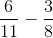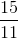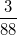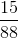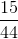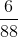Explanation:

Find the common denominator by multiplying both denominators together.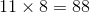Multiply the top with what was multiplied on the bottom to get the denominator. Simplify the fraction.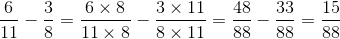### Example Question #41 : Algebraic Fractions

Evaluate: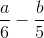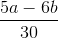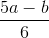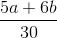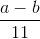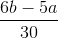Explanation:

Multiply the denominators together to obtain the least common denominator, or LCD.  Then multiply the numerators with what was multiplied on the denominator to get the LCD.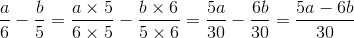The answer is:### Example Question #43 : Algebraic Fractions

Simplify: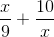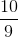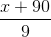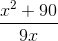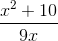Explanation:

Find the common denominator and simplify.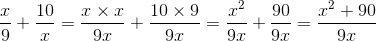### Example Question #43 : Algebraic Fractions

Solve: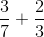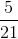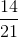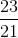Explanation:

In order to evaluate this fraction, we will need to find the least common denominator.  Multiply both denominators together.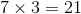Convert both fractions with the similar common denominator.  Multiply the numerator with what was multiplied to get the denominator.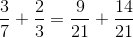Add the numerators on the right side.  The denominators will stay the same.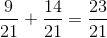The answer is:### Example Question #21 : How To Evaluate A Fraction

In a sample of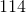students,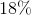of them liked baseball. If there are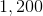students in the entire school, how many students are likely to like baseball?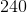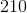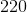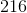Explanation:

To answer this question, we need to convertto a decimal.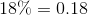. Now we multiplyby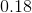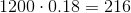.

### Example Question #1 : How To Evaluate A Fraction

Mary walked to school at an average speed of 2 miles per hour and jogged back along the same route at an average speed of 6 miles per hour. If her total traveling time was 1 hour, what was the total number of miles in the round trip?Explanation:

Since Mary traveled 3 times as quickly coming from school as she did going to school (6 miles per hour compared to 2 miles per hour), we know that Mary spent only a third of the time coming from school as she did going. If x represents the number of hours it took to get to school, then x/3 represents the number of hours it took her to return.

Knowing that the total trip took 1 hour, we have:

x/3 = 1

3x/3 + 1x/3 = 1

4x/3 = 1

= 3/4

So we know it took Mary 3/4 of an hour to travel to school (and the remaining 1/4 of an hour to get back).

Remembering that distance =  rate * time, the distance Mary traveled on her way to school was (2 miles per hour) * (3/4 of an hour) = 3/2 miles. Furthermore, since she took the same route coming back, she must have traveled 3/2 of a mile to return as well.

Therefore, the the total number of miles in Mary's round trip is 3/2 miles + 3/2 miles = 6/2 miles = 3 miles.

1 3 Next →

### All SAT Math Resources